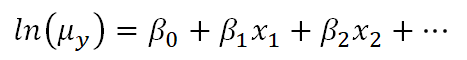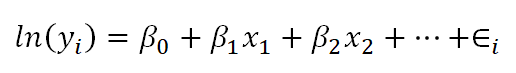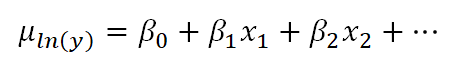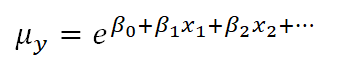# log transformation

### Count Models: Understanding the Log Link Function

November 12th, 2020 by

When we run a statistical model, we are in a sense creating a mathematical equation. The simplest regression model looks like this:

Yi = β0 + β1X+ εi

The left side of the equation is the sum of two parts on the right: the fixed component, β0 + β1X, and the random component, εi.

You’ll also sometimes see the equation written (more…)

### The Difference Between Link Functions and Data Transformations

September 24th, 2018 by

Generalized linear models—and generalized linear mixed models—are called generalized linear because they connect a model’s outcome to its predictors in a linear way. The function used to make this connection is called a link function. Link functions sounds like an exotic term, but they’re actually much simpler than they sound.

For example, Poisson regression (commonly used for outcomes that are counts) makes use of a natural log link function as follows:Clearly, there is not a direct linear relationship of the x variables to the average count, but there is a “sort of linear” relationship happening: a function of the mean of y is related to a linear combination of x variables. In other words, the linear model has now been generalized to a bigger type of situation.

This can lead to confusion, though, because on the surface it looks very similar to what happens when we transform the dependent variable in a linear model, like a linear regression.

The key thing to understand is that the natural log link function is a function of the mean of y, not the y values themselves.

### Transformations of Y

Below is a linear model equation where the original dependent variable, y, has been natural log transformed. That is, the natural log has been taken of each individual value of y, and that is being used as the dependent variable.The linear model with the log transformation is providing an equation for an individual value of ln(y). We could also write it as follows, where we are modeling the mean of ln(y) (note the error term is no longer present):This makes the difference a bit clearer. When we transform the data in a linear model, we are no longer claiming that y is normally distributed around a mean, given the x values — we are claiming that our new outcome variable, ln(yi), is normally distributed.

In fact, we often make this transformation specifically because the values of y do not appear to be normally distributed around their average.

In the case of the Poisson model, however, the link function does not change the distribution of the actual observations in some way to make them something other than Poisson distributed. Instead, the link function defines the relationship of the x variables directly to the mean of the Poisson distributed y. The individual observations then vary around this expected value accordingly.

### The mean of the log is not the log of the mean

As you may know, if you have used this kind of data transformation in a linear model before, you cannot simply take the exponent of the mean of ln(y) to get the mean of y.

You might be surprised to know, though, that you can do this with a link function. If you have specific values of your x variables, you can calculate the predicted average count, μy based on those x values by inversing the natural log:This ability to back-transform means (and regression coefficients) to a more intuitive scale is part of what makes generalized linear models so useful.

### Member Training: Using Transformations to Improve Your Linear Regression Model

March 5th, 2018 by

Transformations don’t always help, but when they do, they can improve your linear regression model in several ways simultaneously.They can help you better meet the linear regression assumptions of normality and homoscedascity (i.e., equal variances). They also can help avoid some of the artifacts caused by boundary limits in your dependent variable — and sometimes even remove a difficult-to-interpret interaction.

### Member Training: A Primer on Exponents and Logarithms for the Data Analyst

January 2nd, 2018 by

Ah, logarithms. They were frustrating enough back in high school. (If you even got that far in high school math.)And they haven’t improved with age, now that you can barely remember what you learned in high school.

And yet… they show up so often in data analysis.

If you don’t quite remember what they are and how they work, they can make the statistical methods that use them seem that much more obtuse.

So we’re going to take away that fog of confusion about exponents and logs and how they work. (more…)